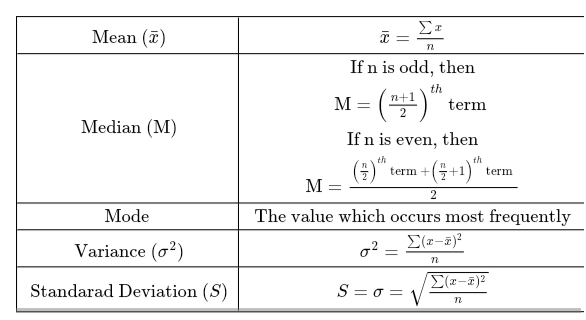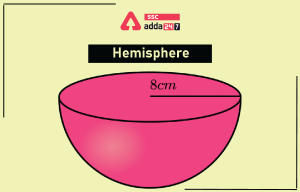Latest SSC jobs   »   SSC Calendar 2022   »   Elementary Statistics

# Elementary Statistics – Problems, Questions, Topics, Types and Formulas

## Elementary Statistics

Elementary Statistics is a branch of mathematics that deals with the collection, analysis, interpretation, and presentation of data (information) to get the required results. In elementary statistics, we study huge data and organize it in the required form. Elementary statistics are used in various applications of education, research, business, politics, economy, etc. Elementary statistics is useful in the observation of data and making a proper analysis of the data to get the desired result. The elementary of statistics makes the analysis of data easy and effective using formulas and methods. The Elementary of statistics helps to represent high data in the required form to get the desired result. The elementary of statistics has many applications in science, and technology, research, analysis, surveys, etc.

In our daily life, we see elementary statistics and its applications in various ways. In newspapers, magazines, television, and business sectors, elementary statistics are used to represent the analysis of data. The elementary of statistics is used to represent data including ages of people, the salary of employees, consumption of any particular commodity, population, selling of products, profit and loss, etc.

## Elementary Statistics Problems

Elementary Statistics problems help you to understand the concepts well and use formulas and trucks to solve the questions of statistics easily. It’s an important section of mathematics asked in almost all government exams. The elementary statistics problems will help you to teach the tech way of solving them most easily.

## Elementary Statistics Questions

Elementary statistics questions include meaning, mode, variance, standard deviation, percentage comparison, etc. The students must practice more and more elementary statistics questions to improve their accuracy and speed. The questions of elementary statistics help to understand the analysis and representation of data. Some important questions about elementary statistics are mentioned below.

Que.1: Find the mode of the following marks out of 10 obtained by 15 students. 4, 3, 5, 6, 2, 5, 4, 7, 8, 9, 2, 4, 5, 6, 4 in a mock test of Mathematics.

Solution: On arranging the obtained marks of students in ascending order 2, 2, 3, 4, 4, 4, 4, 5, 5, 5, 6, 6, 7, 8, 9 Since, 4 occurs four times in the series

So, the mode of given data is 4

Que.2: The marks obtained by 13 students in an examination are 55, 65, 72, 94, 64, 68, 54, 62, 58, 75, 80, 82, and 60. Find the median for the given data.

Solution: Arranging the marks obtained by students in ascending order 54, 55, 58, 60, 62, 64, 65, 68, 72, 75, 80, 82, 94.

Since the total number of given observations is 13 (odd)

So the median will be 7th observation = 65

## Elementary Statistics Topics

Elementary statistics includes many topics and questions are asked them. The elementary topics include types of statistics, mean, median, mode, variance, standard deviation, etc. We are going to discuss all such topics of elementary statistics in detail which are helpful for the students in their upcoming exams.

### Mean

The mean is defined as the average of data and can be calculated easily using the formula mentioned here. Mean = sum of observations/ Total number of observations

### Median

The middlemost term of the observation of given data is called the Median. We can find the median data with the help of some steps listed below.

1.  Firstly, you need to arrange the given data in ascending or descending order
2.  Then count the total number of observation
3.  When the number of observations (n) is odd, the median is the value of this term
4.  When the number of observations (n) is even, the median is the value of the term at an even place

### Mode

The mode is the value of the observation which occurs most times and the mode of the data is denoted by M0

## Elementary Statistics Types

Elementary Statistics is a branch of mathematics in which the collection, analysis, observation, interpretation, and representation of data are performed to get the desired result. The elementary statistics are of the following two types as mentioned below.

• Descriptive Statistics – Descriptive statistics is an important type of statistics focused on the collection, organizing, analysis, and representation of huge data to transform it into a meaningful form. Examples: a teacher wants to calculate a percentage of marks obtained by a whole class of 80 students, an entrepreneur wants to calculate a salary provided to a total of 150 employees of the company.
• Inferential Statistics –  Inferential statistics is a type of statistics that deals with the generalization and analysis of data to get conclusions about a population. It’s a process of obtaining data from large information and interpreting it into smaller groups. For example, a manager wants to predict the profit of the company by analyzing previous data for the next 4 years, and a principal of the school wants to calculate the performance of students in the upcoming board examinations based on the previous results.

## Elementary Statistics Formulas

The formulas of elementary statistics help to calculate the statistics terms easily. The important statistical formulas are listed below.Where, x = Given observations

X bar = mean

n = total number of observations

### Elementary Statistics: FAQs

Que.1 What is elementary statistics?

Ans – Elementary statistics is a branch of mathematics that deals with the collection, analysis, interpretation, and representation of data in a useful form.

Que.2 What is the mode?

Ans – Mode in statistics is defined as the terms that occur most frequently times in an observation.

Que.3 Define the mean of the observation?

Ans – Mean of the observation is defined as the average or term obtained by dividing the sum of terms by the total number of terms.

Que.4 Age of students is given as 14, 15, 16, 15, 15, 17, 15, 18. Find the mode.

Solution: Given ages {14, 15, 16, 15, 15, 17, 15, 18}

Here only term 15 repeats itself four times. So the mode is 15

Mode = {15}

#### Congratulations!General Awareness & Science Capsule PDF•RRB NTPC CBT 2 Syllabus 2022, Topics, Su...
•What is Frequency Polygon? Statistics, G...
•Histogram Definition, Graph, Chart, Form...
•Hemisphere Definition, Volume, Formula, ...